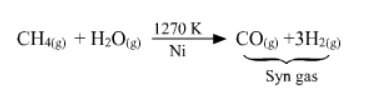# What do you understand by the terms:

Question:

What do you understand by the terms:

(i) hydrogen economy

(ii) hydrogenation

(iii) ‘syngas’

(iv) water-gas shift reaction

(v) fuel-cell ?

Solution:

(i) Hydrogen economy

Hydrogen economy is a technique of using dihydrogen in an efficient way. It involves transportation and storage of dihydrogen in the form of liquid or gas.

Dihydrogen releases more energy than petrol and is more eco–friendly. Hence, it can be used in fuel cells to generate electric power. Hydrogen economy is about the transmission of this energy in the form of dihydrogen.

(ii) Hydrogenation

Hydrogenation is the addition of dihydrogen to another reactant. This process is used to reduce a compound in the presence of a suitable catalyst. For example, hydrogenation of vegetable oil using nickel as a catalyst gives edible fats such as vanaspati, ghee etc.

(iii) Syngas

Syngas is a mixture of carbon monoxide and dihydrogen. Since the mixture of the two gases is used for the synthesis of methanol, it is called syngas, synthesis gas, or water gas.

Syngas is produced on the action of steam with hydrocarbons or coke at a high temperature in the presence of a catalyst.

$\mathrm{C}_{\mathrm{n}} \mathrm{H}_{2 \mathrm{n}+2}+n \mathrm{H}_{2} \mathrm{O} \frac{1270 \mathrm{~K}}{\mathrm{Ni}} \rightarrow n \mathrm{CO}+(2 \mathrm{n}+1) \mathrm{H}_{2}$

For example :(iv) Water shift reaction

It is a reaction of carbon monoxide of syngas mixture with steam in the presence of a catalyst as:

$\mathrm{CO}_{(g)}+\mathrm{H}_{2} \mathrm{O}_{(g)} \frac{673 \mathrm{~K}}{\text { Calalyst }} \mathrm{CO}_{2(g)}+\mathrm{H}_{2(g)}$

This reaction is used to increase the yield of dihydrogen obtained from the coal gasification reaction as:

$\mathrm{C}_{(s)}+\mathrm{H}_{2} \mathrm{O}_{(g)} \longrightarrow \mathrm{CO}_{(g)}+\mathrm{H}_{2(g)}$

(v) Fuel cells

Fuel cells are devices for producing electricity from fuel in the presence of an electrolyte. Dihydrogen can be used as a fuel in these cells. It is preferred over other fuels because it is eco-friendly and releases greater energy per unit mass of fuel as compared to gasoline and other fuels.